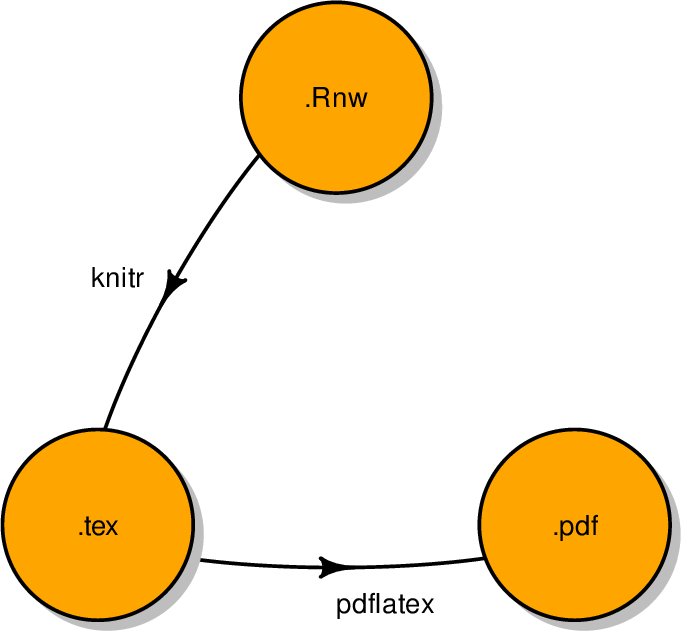Data Science Desktop Survival Guide by Graham WilliamsDesktop Survival Project Home Preface Data Science Introducing R R Constructs R Tasks R Strings R Read, Write, and Create Data Template Data Exploration Data Wrangling Data Visualisation Statistics ML Template ML Scenarios ML Activities ML Applications ML Algorithms Cluster Analysis Decision Trees Computer Vision Graph Data Privacy Literate Data Science Coding with Style Resources Bibliography Index

# Load package from the local library into the R session.

library(diagram)

# Identify some names for the nodes of the flowchart.

names   <- c(".Rnw", ".tex", ".pdf")

# Set up the connectivity data.

connect <- c(0,       0,          0,
"knitr", 0,          0,
0,       "pdflatex", 0)

# Constract a connectivity matrix and plot the flowchart.

matrix(nrow=3, ncol=3, byrow=TRUE, data=connect) %>%
plotmat(pos=c(1, 2), name=names, box.col="orange")There are many more possibilities provided by diagram and if interested you can explore demo(plotmat) and demo(plotweb).